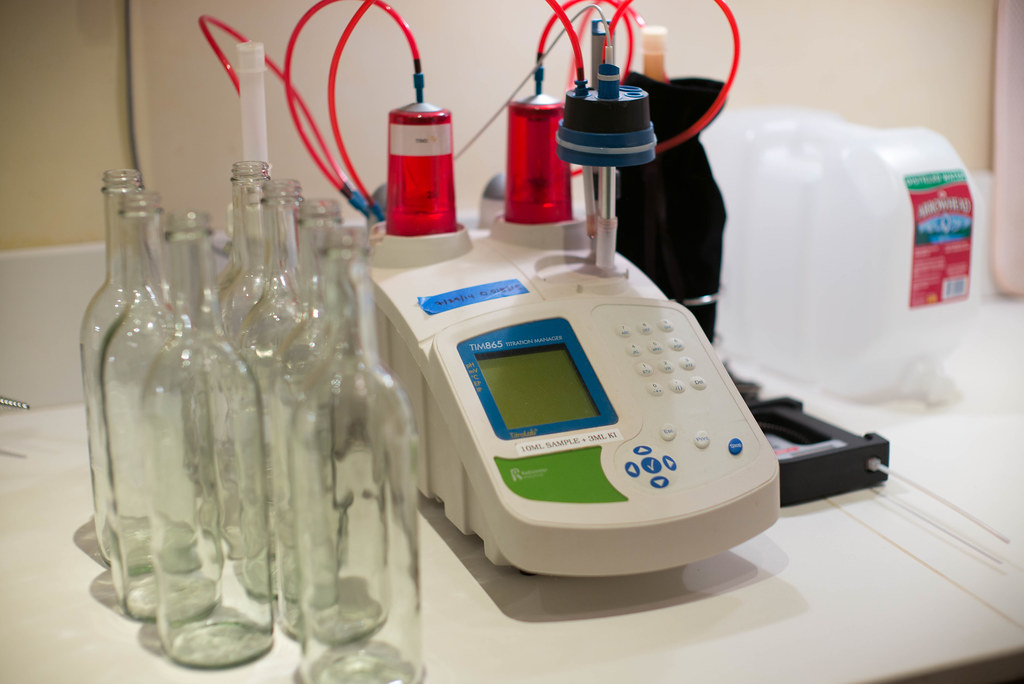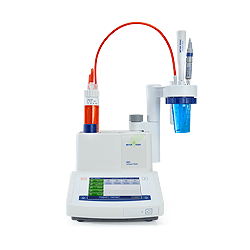Titrator

# Definition, How it Works, and Types of Acid-Base Titration

Created By: Hyprowira | Published Date: 18 May 2021 | Last Modified: 18 May 2021

If you studied Chemistry in high school, you may be familiar with the term acid-base titration. Titration itself is used to analyze quantitative chemistry, which is a common laboratory method. Generally, this method is used to determine what the unknown titrant concentration looks like. The titration process is basically used in calculating the properties of a solution

## Definition of Acid-Base Titration

Acid-base titration is a chemical analysis method that is often used quantitatively in the laboratory. This procedure is used to determine the level of an acid-base or polarity based on a neutralization reaction.

Acid-base titration functions to determine reactants and concentration. You need to know, there is a titrant as a known solution and a titrant for which the titration will be determined in the ongoing titration process.

To carry out the titration process, the equipment that is often used is the titration meter. As you probably know, the polarity will show the number of moles of the substance dissolved per liter of solution and can be used to determine the dilution of a solution.

It is also important for you to know some of the terms in acid-base titration, including:

1. Pentiter. In determining the polarity of an acid-base, a pentiter is the substance that titrates
2. The end point of the titration. This is the point when the acid-base indicator changes color
3. Equivalence point. This is the point at which the acid-base is completely exhausted

## How Acid-Base Titration Works

Acid-base solutions in chemistry are often used to determine the pH value or the degree of acidity of a solution. How it works, substances that are acidic will be titrated using an alkaline solution whose concentration has been known. This process can also be applied the other way around, namely substances that are alkaline are titrated using an acidic solution with known concentrations.

Because it applies the principle of the acid-base reaction, acid-base titration will produce a neutralizing reaction in the form of salt and water with a neutral pH (7) when acidic and basic compounds are reacted. In addition to determining the molality of a solution whose concentration is unknown, acid-base titration is also used to determine the mass percentage of solutes in a particular solution.

In addition, this method can be used to find the percent purity of a chemical element. Can also be used to perform tests for buffering activities. This acid-base titration is an application used for the process of testing blood sugar, nutrition, pregnancy tests, wastewater analysis, and laboratory water testing.

The steps for how the acid-base titration works are as follows:

1. If you want to determine a compound which is not known in levels, you first need to know the acidic nature of the substance by measuring its pH.
2. After knowing the properties of the substance whose levels are to be measured, then let's determine the solution that will be used in the titration process of the substance. You can use an acid or base solution.
3. A glass device called a burette is generally used in the titration process. A burette is a tube that has been calibrated vertically and will be suspended using a plug right at its base.
4. Burette is used to see the change in the volume of the titrant before and after it is used for titration. The more widely used titrant volume indicates a higher concentration level.
5. In this titration process, the burette helps regulate the flow of liquid into the flask. When the liquid flows into the flask, there will be a color change, so the pH indicator will turn pink or methyl orange.
6. Then, a known solution is added to the substance being tested slowly to obtain a neutralizing reaction.
7. If the mixed solution has a neutral pH, that is a sign that all sample substances have reacted to the solution used in the titration process.

## Types of Acid-Base Titrations

For its type, acid-base titrations are of two types, namely acidimetry and alkalimetry. Acid-base titration of the acidimetric type is a way of calculating the concentration of an alkaline solution using an acid standard solution. Meanwhile, alkalimetric acid-base titration is a way of calculating the determination of the concentration of the acid solution using a standard base solution.

That's the information about acid-base titration that you need to know. If you need the right tools for analysis, you can use the karl fischer mettler toledo which you can find at Hyprowira. Hopefully the information about acid-base titrations above can help you!

Also read: 4 Factors Affecting PH

18815 views

### Other Articles